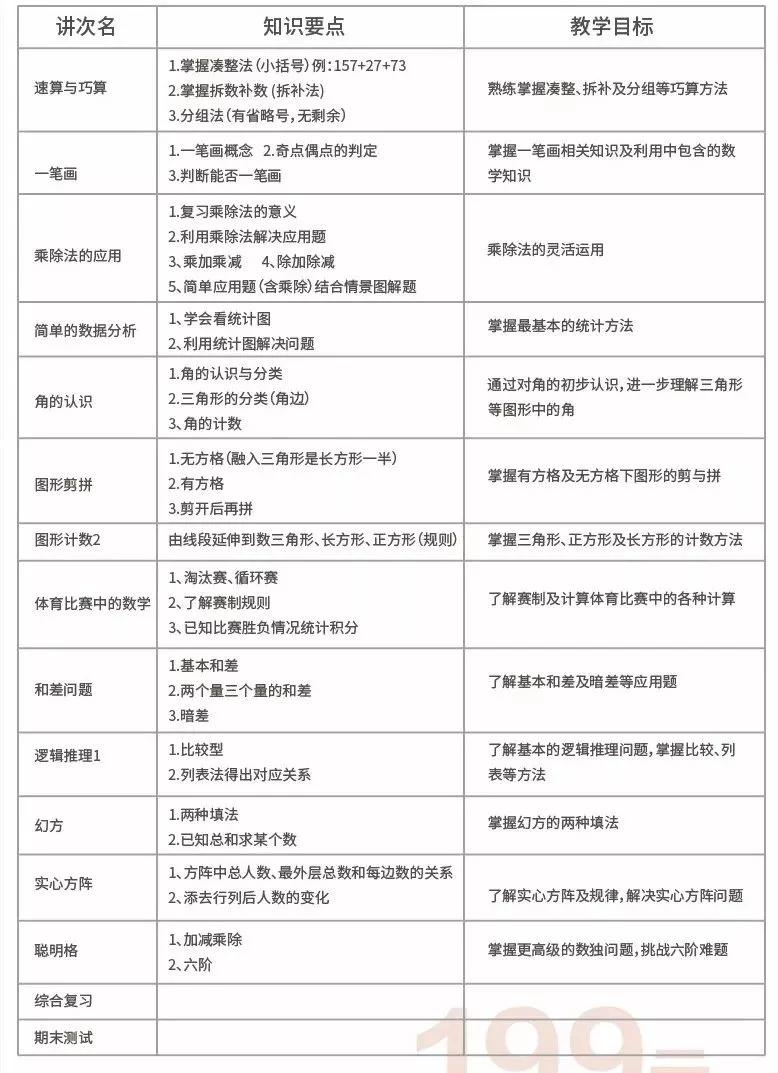### 小学二年级数学知识点归纳，暑假提前学，开学变学霸！

导读：本文是来自吉林省临江市的网友投稿，由分数乘法课件编辑发布关于小学二年级数学知识点归纳，暑假提前学，开学变学霸！

的内容介绍

原标题：小学二年级数学知识点归纳，暑假提前学，开学变学霸！知识点概括总结

1.长度单位：是指丈量空间距离上的基本单元，是人类为了规范长度而制定的基本单位。其国际单位是“米”(符号“m”)，常用单位有毫米(mm)、厘米(cm)、分米(dm)、千米(km)等等。长度单位在各个领域都有重要的作用。

2.米：国际单位制中，长度的标准单位是“米”，用符号“m”表示。

3.分米：分米(dm)是长度的公制单位之一，1分米相当于1米的十分之一。

4.厘米：厘米,长度单位。简写(符号)为:cm.

有关厘米的单位转换: 1厘米=10毫米=0.1分米=0.01米=0.00001千米。

5.毫米：英文缩写MM(或mm、㎜)

进率关：1毫米=0.1厘米;

6.进位：加法运算中，每一数位上的数等于基数时向前一位数进一。

以个位向十位进位为例：基数为10(2进制的基数是2，类推)，个位这个数位上的数量达到了10的情况下，则个位向前一位进1，成为一个十。

在十进制的算法中，个位满十，在十位中加1;十位满十，在百位中加一。

7.不退位减：减法运算中不用向高位借位的减法运算。例：56-22=34。6能够减去2，所以不用向高位5借位。

8.退位减：减法运算中必须向高位借位的减法运算。例：51-22=39.

1不能够减去2，所以必须向高位的5借位。

9.连加：多个数字连续相加叫做连加。例如：28+24+23=85.

10.连减：多个数字连续相减叫做连减。例如：85-40-26=19.

11.加减混合：在运算中既有加法又有减法的运算。例如：67-25+28=70。

12.角：具有公共端点的两条不重合的射线组成的图形叫做角。这个公共端点叫做角的顶点，这两条射线叫做角的两条边。

符号 ：∠

13.乘法算式中各数的名称：是指将相同的数加法起来的快捷方式。其运算结果称为积。

“×”是乘号，乘号前面和后面的数叫做因数，“=”是等于号，等于号后面的数叫做积。

10(因数) ×(乘号) 200(因数)=(等于号) 2000(积)

14.1—6的乘法口诀

1×1=1

1×2=22×2=4

1×3=32×3=63×3=9

1×4=42×4=83×4=124×4=16

1×5=52×5=103×5=154×5=205×5=25

1×6=62×6=123×6=184×6=245×6=306×6=36

15.7——9的乘法口诀

1×7=72×7=143×7=214×7=285×7=356×7=427×7=49

1×8=82×8=163×8=244×8=325×8=406×8=487×8=568×8=64

1×9=92×9=183×9=274×9=365×9=456×9=547×9=638×9=729×9=81

扩展资料：

1.角的动态定义

一条射线绕着它的端点从一个位置旋转到另一个位置所形成的图形叫做角。所旋转射线的端点叫做角的顶点，开始位置的射线叫做角的始边，终止位置的射线叫做角的终边

2.角的种类

角的大小与边的长短没有关系;角的大小决定于角的两条边张开的程度，张开的越大，角就越大，相反，张开的越小，角则越小。在动态定义中，取决于旋转的方向与角度。角可以分为锐角、直角、钝角、平角、周角、负角、正角、优角、劣角、0角这10种。以度、分、秒为单位的角的度量制称为角度制。此外，还有密位制、弧度制等。

锐角：大于0°，小于90°的角叫做锐角。

直角：等于90°的角叫做直角。

钝角：大于90°而小于180°的角叫做钝角。

负角：按照顺时针方向旋转而成的角叫做负角。

正角：逆时针旋转的角为正角。

0角：等于零度的角。

余角和补角：两角之和为90°则两角互为余角，两角之和为180°则两角互为补角。等角的余角相等，等角的补角相等。

对顶角：两条直线相交后所得的只有一个公共顶点且两个角的两边互为反向延长线，这样的两个角叫做互为对顶角。两条直线相交，构成两对对顶角。互为对顶角的两个角相等。

还有许多种角的关系，如内错角,同位角，同旁内角(三线八角中，主要用来判断平行)!

3.乘法的运算定律

整数的乘法运算满足：交换律，结合律， 分配律，消去律。

随着数学的发展， 运算的对象从整数发展为更一般群。

乘法交换律：a×b=b×a

乘法结合律：(a×b)×c=a×(b×c)

乘法分配律：(a+b)×c=a×c+b×c

二年级下册

知识点概括总结

1.表内除法的知识点：

(1)理解平均分的意义。会根据表内乘法，计算简单的除法。

(2)会用乘法口诀求商。

(3)根据乘除法的意义解决一些简单的乘除法应用题。

(4)被除数÷除数=商 被除数÷商=除数 除数×商=被除数

2.除法：是四则运算之一,已知两个因数的积与其中一个因数，求另一个因数的运算，叫做除法。

3.除法的性质

一个数连续除以几个数，等于这个数除以那几个数的乘积，就是除法的性质。有时可以根据除法的性质来进行简便运算。如：300÷25÷4=300÷(25×4)

4.除法公式

(1)被除数÷除数=商

(2)被除数÷商=除数

(3)除数×商=被除数

5.被除数

除法运算中被另一个数所除的数,如24÷8=3,其中24是被除数

6.除数：在除法算式中，除号后面的数叫做除数。

例：8÷2=4则2为除数。8为被除数。除数不能为0，否则没有意义。

7.商：在一个除法算式里，被除数÷除数=商+余数，进而推导得出：商×除数+余数=被除数。

8.完全商

当数a除以数b(非0)能除得尽时，这时的商叫完全商。如:9÷3=3，3就是完全商。

9.不完全商

如果数a除以数b(非零)除不尽，得到的商就是不完全商。如：10÷3=3......1，这里的3就是不完全商。

10.被除数和商的关系

被除数扩大(缩小)n倍，商也相应的扩大(缩小)n倍。

除数扩大(缩小)n倍，商相应的缩小(扩大)n倍)。

11.2—6的乘法口诀

2×2=4

2×3=63×3=9

2×4=83×4=124×4=16

2×5=103×5=154×5=205×5=25

2×6=123×6=184×6=245×6=306×6=36

12.直角：几何原本中的定义：当一条直线和另一条横的直线交成的邻角彼此相等时，这些角的每一个被叫做直角，而且称这一条直线垂直于另一条直线。

一个直角等于90度，符号：Rt∠

13.几何中的锐角：大于0°小于90°(直角)的角。

两个锐角相加不一定大于直角，但一定小于平角。

14.钝角：钝角大于直角(90°)小于平角(180°)的角叫做钝角。

15.平移：平移是指在平面内，将一个图形上的所有点都按照某个方向作相同距离的移动，这样的图形运动叫做图形的平移运动，简称平移。平移不改变图形的形状和大小。平移可以不是水平的。

16.旋转：在平面内，把一个图形绕点O旋转一个角度的图形变换叫做旋转，点O叫做旋转中心，旋转的角叫做旋转角，如果图形上的点P经过旋转变为点Pˊ，那么这两个点叫做这个旋转的对应点。

17.旋转的性质

(1)对应点到旋转中心的距离相等。

(2)对应点与旋转中心所连线段的夹角等于旋转角。

(3)旋转前、后的图形全相等。

18.旋转的三要素

(1)旋转中心;

(2)旋转方向;

(3)旋转角度。

注意：三要素中只要任意改变一个，图形就会不一样。

旋转变换是由一个图形改变为另一个图形，在改变过程中，原图上所有的点都绕一个固定的点换同一方向，转动同一个角度

19.表内除法的知识点：

(1)理解平均分的意义。会根据表内乘法，计算简单的除法。

(2)会用乘法口诀求商。

(3)根据乘除法的意义解决一些简单的乘除法应用题。

(4)被除数÷除数=商被除数÷商=除数 除数×商=被除数

20.7、8、9的乘法口诀

7×7=49

7×8=568×8=64

7×9=638×9=729×9=81

21.万以内的数的认识

100=10个10(10个10相加的结果等于100)

1000=10个100(10个100相加的结果等于1000)

22.克

克为质量单位，符号 g，相等于千分之一千克。一克的重量大约相于一立方厘米水在室温的质量，大约有一个万字夹的质量。

1 吨=1,000,000 克 (一百万克)

1 公斤(1千克)=1,000 克 (一千克)

1 市斤=500克 (1 克=0.002市斤)

1 毫克=0.001 克 (1克=1000毫克)

1 微克=0.000 001 克 (1克=1000000微克)

1 纳克=0.000 000 001 克(1克=1000000000纳克)

23.千克

千克：(符号kg或㎏)为国际单位制中量度质量的基本单位，千克也是日常生活中最常使用的基本单位之一。

倘若孩子心思没放在学习上，

任凭家长们怎样努力都是无济于事的！

那你有没有方法让孩子喜欢课堂呢？

当然有，孩子喜欢老师，就喜欢课堂！

喜欢课堂，就能出成绩！

寓教于乐得过程，让孩子爱上课堂！

触类旁通

学生对于数学学习呈现明显不同的两种状态，擅长逻辑思维的孩子总能从中洞察种种规律，而另外一部分孩子就显得很吃力。数学教学工作旨在带领孩子探寻数学的乐趣并能从中掌握独有的解题思维，怎样的课程才最有效果呢？举一反三、触类旁通才是我们的目的，一个学科的积淀能渗透到种种学科，这才是真正的成长！

激发兴趣，培养习惯

新舟针经过大量调研和反复研讨，完善了一套非常高效的数学教学体系。课程旨在激发兴趣，我们通过有趣的讲义设计、丰富的课堂活动设计让孩子们探寻学习乐趣。另一方面，还将从培养习惯入手，从课前、课中、课后关注孩子预习习惯、笔记习惯、复习习惯、反思习惯等，让孩子因习惯而优秀。

2019暑假，新舟开放数学暑期名师班，仅针对新二年级，新舟中小学12家校区同步开放，数学教学优势

1、立足校内，顶层设计严格遵循课标、课本要求进行设计；

2、精准定位小升初，拆解小升初试卷，1-4专项基础知识学会，5年级专项突破+真题模拟；

3、重原理探究，通过课堂活动让孩子感知原理形成的过程，进而归纳、总结原理；

4、重效果，根据学情，由浅入深，重课上吸收，抓课后落实！

暑期二年级数学课程大纲责任编辑：